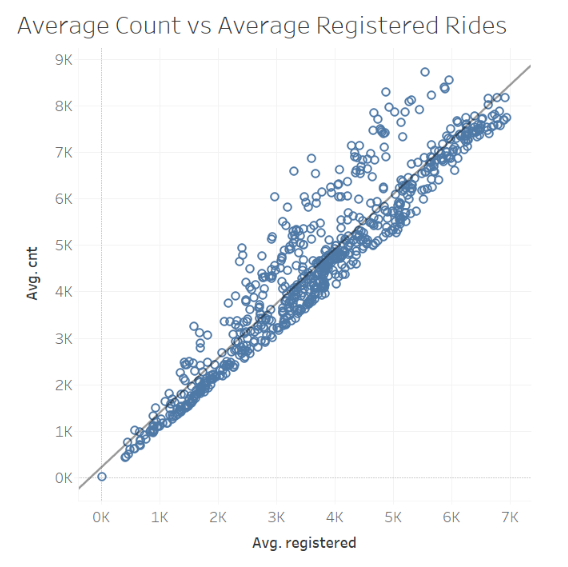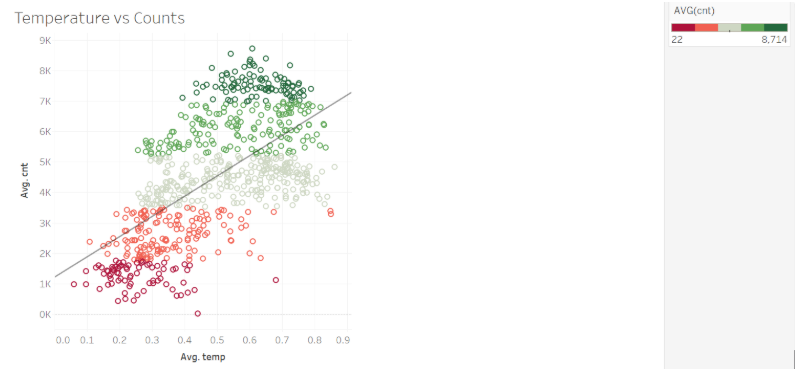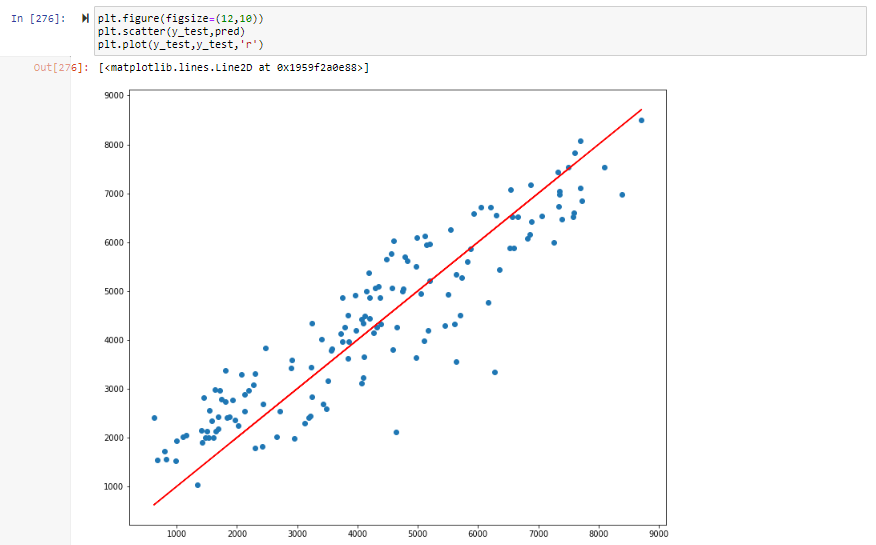# Artificial Neural Networks, Part 3 — Implementing Neural Networks with Keras and TensorflowPhoto by Max Duzij on Unsplash

In the previous posts of this series, we covered the following topics —

This post will cover the implementation of a basic neural network using Tensorflow and Keras.

The problem is based on the Bike Sharing Dataset available on UCI Machine Learning repository and can be found here —

Bike Sharing Dataset

There are two datasets provided in the zip file, daily.csv, and hour.csv.

In this problem, I have used the daily.csv file which is a rollup of the hour.csv file.

The problem involves predicting the count of rental bikes including per day.

Let us start with the implementation steps —

The first step starts with importing the required libraries and reading the datasets

Now, that we have imported the CSV files as pandas DataFrames, let's look into the shape and the details of the available columns.

There are 731 records, and 16 columns including our target variable “cnt”, which is a count of daily rental bikes. The details for every column can be found on the UCI dataset link provided above.

Looking at the first few rows of the daily dataset —

We can see that the values of the columns season,mnth, weekday,workingday, and weathersit are provided as numbers and hence are treated as numeric columns. It would be beneficial if we convert these as categorical for some visualizations for EDA.

We will first start by plotting the correlation as a heatmap.

As a first impression of the values, it can be inferred that the variables are not very highly correlated with the target, except for the variable ‘registered’ which has a value of 0.95.

For EDA, lets us start with the univariate visualizations.

The target variable does not contain any negative values or any outliers.

Moving on to independent variables,

1. Holiday — 0 (No), 1 (Yes)

2. Working day — 0 (No), 1 (Yes)

3. Weathersit —

Plotting the target variable with the independent variable —

The average usage is higher in the months, 6–9 i.e. June — September.

Again more usage is season 2 and 3 i.e. Spring and Summer.

Identifying the relationship and behavior between the numeric columns against the target.As we saw earlier as well, there is a high correlation between the Registered and total rides.More usage during mid-temperature ranges

Clearly, atemp and temp variables are highly correlated. We can drop one of the variables.

Based on the EDA, we will drop the following variables —

dteday,instant,atemp,yr,registered

After EDA, we will work on setting up our dataset for training and testing the model.

Encoding categorical data —

We can also use sklearn’s OneHotEncoder library, but here I am using pandas getdummies() and a small code snippet to keep the columns the same in both train and test sets after encoding.

Scaling values to stay in the same range of 0 and 1—

Importing libraries for building the network

We are importing the Sequential class to create the model which will be stacked into layers.

The Dense class will create a deeply connected neural network layer. This layer would perform the operation on the input, apply the activation function, and pass on the results to the next layer.

Building the network —

The first layer is a dense layer with 34 nodes, this is basically the input layer and the value 34 comes from the number of features in our dataset after the processing.

The second, third, and fourth layers are the hidden layers and contain 34,34 and 10 nodes respectively. Finally, we add a single node, which is our output. The output for us is a numeric value else, in a classification case, it will be the number of classes in the target.

Compiling the model —

Here the loss function is MSE, Mean Squared Error. We will be looking to minimize this. It will be achieved using Adam optimizer. An optimizer works towards minimizing the value of our loss function.

Finally, fitting the model on our training data. We have also passed the test data as a validation set. The process will continually compute the loss of training and test set. The number of epochs is 1000, which I have chosen arbitrarily. We can leverage methods like early stopping which will stop the training as the minimum value of loss function is used. The batch size is 64, given the number of records is less in our training and test sets.

Visualizing the loss values —

We can see that the value of validation loss and training loss became minimum on the 400th epoch. We can still look into more number of epochs to see when the validation loss starts increasing and we start overfitting. But for this blog, we will stick with the 1000 epochs.

Lets quickly dive into the predictions and calculating the metrics.

The mean squared error is approx 70k with the mean absolute error at 703. Our model is able to explain 83% of the variance. Which is good, but we can still improve and get a lower MSE.

Lets plot predicted vs actuals and see how far they areNot really far. Which seems good.

So, this was a quick post on how we can implement a neural network with Keras and Tensorflow.

I hope this helps and I will appreciate any feedback and suggestions on ways to improve the model.

--

--